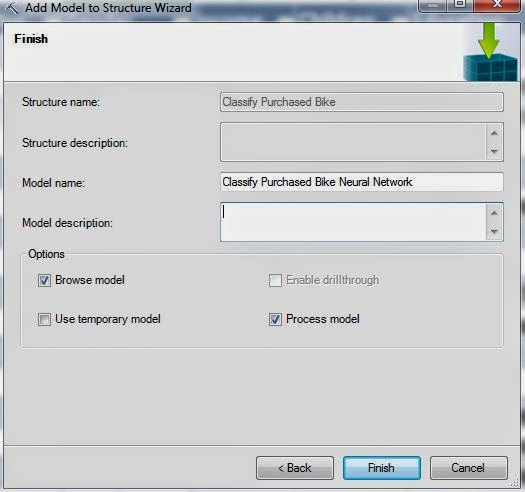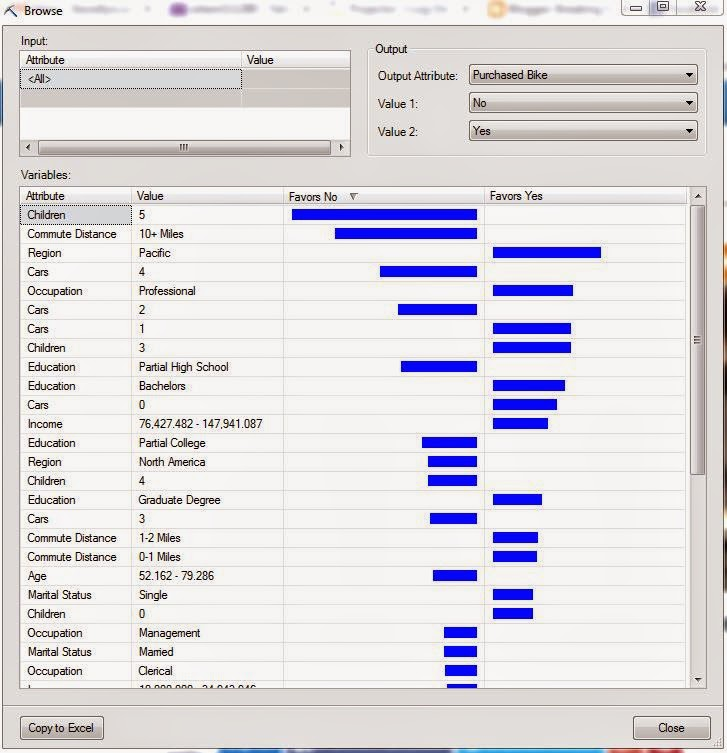## Monday, October 27, 2014

### Data Mining in Excel Part 26: Neural Networks

Today, we'll be talking about the final classification algorithm in the Microsoft Data Mining stack, Neural Networks.  A Neural Network is the pathways that animals (including people) use to develop thoughts and ideas.  Technically, this algorithm builds an Artificial Neural Network in an attempt to emulate that kind of behavior.  In Excel, this algorithm is only accessible through the "Add Model to Structure" button.Add Model to Structure
If you read our post on Logistic Regression, you may recognize some of the concepts here.  The Logistic Regression algorithm is actually a special case of the Neural Network algorithm created by excluding any hidden layers.  For more information about Artificial Neural Networks, read this.  Let's get started.Select Structure
First, we need to select the "Classify Purchased Bike" structure to store our model.Select Algorithm
Then, we need to select the "Microsoft Neural Network" algorithm.  Let's take a look at the parameters.Parameters
The more observant views might notice that the only difference between these parameters and the Logistic Regression parameters is the addition of Hidden Node Ratio.  This makes perfect sense because, as we said earlier, the Logistic Regression algorithm is simply the Neural Network algorithm with no hidden layer, i.e. Hidden Node Ratio = 0.  The Hidden Node Ratio is most useful parameter in this algorithm and can be used to drastically change the model.  For more information about these parameters, read this.  Let's move on.Select Columns
As usual, we want to use all of the columns except ID (_RowIndex) and Purchased Bike to predict Purchased Bike.Create Model
Finally, we need to create the model.  Let's check out the results.Discrimination Report
This Discrimination Report says that we should focus on customers from the Pacific, have a Professional occupation, 1 car, or 3 kids.  You can keep going with that logic for as long as you need.  Just for kicks, let's see what happens if we try a couple different values for Hidden Node Ratio.Discrimination Report (1 HNR)
This model has a Hidden Node Ratio of 1.  This model still has most of the same favorable attributes as the previous one.  That's a good thing because it implies that the model is stable, and therefore good.  Let's try a Hidden Node Ratio of 10.Discrimination Report (10 HNR)
This model looks pretty similar to the others as well.  We should note that we can't suggest stability of the model by using the Discrimination Report.  What we really need to do is see how much the predicted values vary, or don't vary, across the models.  Even better, we could compare the predictions from the Neural Network models to the other classification models we've built previously.  However, we're not quite there yet.  Keep an eye out for our next post where we'll be talking about Accuracy Charts.  Thanks for reading.  We hope you found this informative.

P.S.

We wanted to discuss the Sequence Clustering Algorithm next.  However, we can't add a Sequence Clustering Model to a Structure without a Sequence ID, yet we can't find a way to create a Structure with a Sequence ID.  So, if you know anything about building a Sequence Clustering Model using the Excel interface, let us know in the comments.

Brad Llewellyn
Director, Consumer Sciences
Consumer Orbit
llewellyn.wb@gmail.com
http://www.linkedin.com/in/bradllewellyn